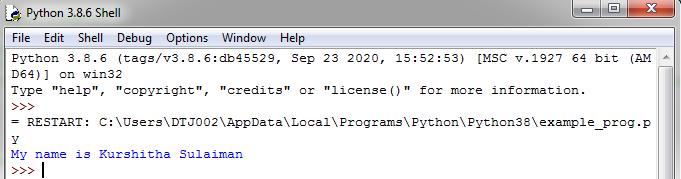## User-defined functions in Python

User-defined functions:

• The users have full scope to implement their own ideas in the function.
• It defined by the users according to their requirements are called user-defined functions.
• Elements of user-defined function:

* Function definition
* Function call or function

Rules:

• Function name must be unique and follows naming rules the same as for identifiers.
• A function can take arguments. It is optional.
• An optional return statement to return a value from the function.
• The function must be called/invoked to execute its code.

Syntax:

1. def function_name(comma separated list of parameters):

statements

function_name(comma separated list of parameters)

Example program:

1. def sum_two_num():#function definition

print("Add of two number is : ",int(a)+int(b))

a,b=input("Enter two values : ").split()

sum_two_num()  #function calling

Output:

Parameters and Arguments in Function:

Parameters:

• Parameters are the value(s) provided in the parenthesis when we
• write function header. These are the values required by the function
• to work.
• The parameter is also known as formal arguments or formal parameter.

Arguments:

• An Argument is a value that is passed to the function when it is called.
•  In other words, arguments are the value(s) provided in the function call / invoke statement.
• Arguments are also known as an actual argument or actual parameter.

Example program:

1. def square(x):#formal argument

print("Cube square value is :",x*x)

a=int(input("Enter any vale for squre root: "))

square(a)#actual argument

Output:

Note:

Where,

• x is a Formal argument
• a is Actual argument

Types of arguments:

There are 4 types of Actual Arguments allowed in Python:

1. Positional arguments

2. Default arguments

3. Keyword arguments

4. Variable length/arbitrary arguments

1. Positional arguments:

1. def div(x,y):

print("Divide of two numbers :",x/y)

a=int(input("Enter first value: "))

b=int(input("Enter second value: "))

div(a,b)

Output:

2. Default arguments

• Sometimes we can provide default values for our positional arguments. In this case, if we are not passing any value then default values will be considered.

1. def sub(x=10,y=7):

print("Subtraction of two value is : ",x-y)

a=int(input("Enter firts number : "))

b=int(input("Enter second number : "))

sub(a,b)

sub()#default value will return

Output:

3. Keyword(Named) Arguments:

• The default keyword gives the flexibility to specify a default value for a parameter so that it can be skipped in the function call if needed. However, still, we cannot change the order of arguments in function call i.e. you have to remember the order of the arguments and pass the value accordingly.
• To get control and flexibility over the values sent as arguments, python offers keyword arguments.
• This allows calling a function with arguments in any order using the name of the arguments.

Rules for combining all three type of arguments:

• An argument list must first contain positional arguments followed by keyword arguments.
• Keyword arguments should be taken from the required arguments.
• You cannot specify a value for an argument more than once.
• Default arguments must not be followed by non-default arguments.

Example program:

1. def mul(x,y=2,z=3):

print("Multiplying of three numbers:",x*y*z)

mul(2)

mul(7,y=3,z=1)

Output:

4. Variable length/arbitrary arguments:

Special Symbols Used for passing arguments:-

1.)*args (Non-Keyword Arguments):

• *args is used to pass the number of argument based on your values.

Example program:

1. def sum(*num):

result = 0

for i in num:

result = result + i

return result

print(sum(1, 2))

print(sum(1, 2, 3))

print(sum(5, 2, 3, 4))

Output:

2.)**kwargs (Keyword Arguments):

Using dictionary:

1. def std(**data):

for key, value in data.items():

print(key," \t: ",value)

std(Roll=4217, Name="Kurshitha", Age=22, Mark=432)

Output:

Using normal variables:

1. def fun(**name):

print("My name is " + name["fname"]+" "+name["lname"])

fun(fname = "Kurshitha", lname = "Sulaiman")

Output: